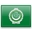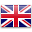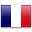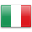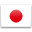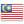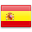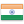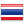VCE Mathematical Methods - Gladstone Park

# VCE Mathematical Methods

https://www.vcaa.vic.edu.au/curriculum/vce/vce-study-designs/mathematicalmethods/Pages/Index.aspx

Is this subject available for acceleration? NO

Mathematical Methods consists of the following areas of study: Functions and Graphs, Algebra, Calculus, and Probability and Statistics.

This link shows a selection of jobs that have some relation to the subject of: Mathematical Methods

##### Units 1 and 2
• Can be taken alone or in conjunction with Year 11 Further Mathematics or Year 11 Specialist Math Units 1 and 2
• Designed for students who are intending to continue with VCE Mathematical Methods Units 3 and 4

##### Units 3 and 4
• Can be taken alone or in conjunction with Year 12 Further Mathematics or Year 12 Specialist Math Units 3 and 4
• Mathematical Methods Units 3 & 4 must be taken if doing Specialist Mathematics Units 3 & 4

A CAS Calculator is required for this subject

##### Skills
• Students will cover the graphical representation of polynomial and power functions
• Students will develop algebraic expression and represent functions, relations, equations and simultaneous equations
• Students should be able define constant and average rates of change
• Students will consider events as either impossible, certain, complementary, mutually exclusive, conditional and independent
• Students will cover simple functional relations, inverse functions and the solution of equations

##### Associated subjects
• Physics
• Chemistry
• Specialist Mathematics
• Further Mathematics
• Economics
• Systems Engineering